# Maxwell's equations facts for kids

Kids Encyclopedia Facts

Maxwell's equations describe how electric charges and electric currents create electric and magnetic fields. They describe how an electric field can generate a magnetic field, and vice versa.

In the 1860s James Clerk Maxwell published equations that describe how charged particles give rise to electric and magnetic force per unit charge. The force per unit charge is called a field. The particles could be stationary or moving. These, and the Lorentz force equation, give everything one needs to calculate the motion of classical particles in electric and magnetic fields.

The first equation allows one to calculate the electric field created by a charge. The second allows one to calculate the magnetic field. The other two describe how fields 'circulate' around their sources. Magnetic fields 'circulate' around electric currents and time varying electric fields: Ampère's law with Maxwell's extension, while electric fields 'circulate' around time varying magnetic fields: Faraday's law.

## Maxwell's Equations in the classical forms

Name Differential form Integral form
Gauss' law: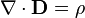$\nabla \cdot \mathbf{D} = \rho$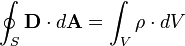$\oint_S \mathbf{D} \cdot d\mathbf{A} = \int_V \rho \cdot dV$
Gauss' law for magnetism
(absence of magnetic monopoles):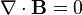$\nabla \cdot \mathbf{B} = 0$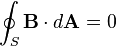$\oint_S \mathbf{B} \cdot d\mathbf{A} = 0$
Faraday's law of induction: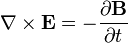$\nabla \times \mathbf{E} = -\frac{\partial \mathbf{B}} {\partial t}$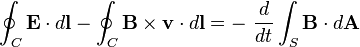$\oint_C \mathbf{E} \cdot d\mathbf{l} - \oint_C \mathbf {B} \times \mathbf{v} \cdot d{\mathbf {l}} = - \ { d \over dt } \int_S \mathbf{B} \cdot d\mathbf{A}$
Ampère's law
(with Maxwell's extension):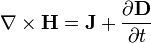$\nabla \times \mathbf{H} = \mathbf{J} + \frac{\partial \mathbf{D}} {\partial t}$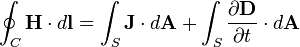$\oint_C \mathbf{H} \cdot d\mathbf{l} = \int_S \mathbf{J} \cdot d \mathbf{A} + \int_S\frac{\partial \mathbf{D} }{\partial t}\cdot d \mathbf{A}$

where the following table provides the meaning of each symbol and the SI unit of measure:

Symbol Meaning SI Unit of Measure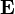$\mathbf{E}$ electric field volt per metre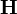$\mathbf{H}$ magnetic field strength ampere per metre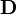$\mathbf{D}$ electric displacement field coulomb per square metre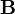$\mathbf{B}$ magnetic flux density
also called the magnetic induction.
tesla, or equivalently,
weber per square metre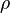$\ \rho \$ free electric charge density,
not counting the dipole charges bound in a material.
coulomb per cubic metre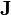$\mathbf{J}$ free current density,
not counting polarization or magnetization currents bound in a material.
ampere per square metre$d\mathbf{A}$ differential vector element of surface area A, with very small
magnitude and direction normal to surface S
square meters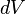$dV \$ differential element of volume V enclosed by surface S cubic meters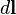$d \mathbf{l}$ differential vector element of path length tangential to contour C enclosing surface c meters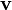$\mathbf{v}$ instantaneous velocity of the line element$d\mathbf{l}$ defined above (for moving circuits). meters per second

and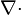$\nabla \cdot$ is the divergence operator (SI unit: 1 per metre),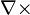$\nabla \times$ is the curl operator (SI unit: 1 per metre).

## Covariant Formulation

There are only two covariant Maxwell Equations, because the covariant field vector includes the electrical and the magnetical field.

Mathematical note: In this section the abstract index notation will be used.

In special relativity, Maxwell's equations for the vacuum are written in terms of four-vectors and tensors in the "manifestly covariant" form. This has been done to show more clearly the fact that Maxwell's equations (in vacuum) take the same form in any inertial coordinate system. This is the "manifestly covariant" form: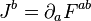$J^ b = \partial_a F^{ab} \,\!$,

and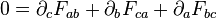$0 = \partial_c F_{ab} + \partial_b F_{ca} + \partial_a F_{bc}$

The second equation is the same as: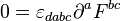$0 = \varepsilon_{dabc}\partial^a F^{bc} \,\!$

Here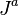$\, J^a$ is the 4-current,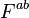$\, F^{ab}$ is the field strength tensor (written as a 4 × 4 matrix),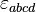$\, \varepsilon_{abcd}$ is the Levi-Civita symbol, and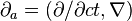$\partial_a = (\partial/\partial ct, \nabla)$ is the 4-gradient (so that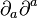$\partial_a \partial^a$ is the d'Alembertian operator). (The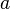$a$ in the first equation is implicitly summed over, according to Einstein notation.) The first tensor equation says the same thing as the two inhomogeneous Maxwell's equations: Gauss' law and Ampere's law with Maxwell's correction. The second equation say the same thing as the other two equations, the homogeneous equations: Faraday's law of induction and the absence of magnetic monopoles.$\, J^a$ can also be described more explicitly by this equation: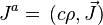$J^a = \, (c \rho, \vec J)$ (as a contravariant vector), where you get$\, J^a$ from the charge density ρ and the current density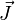$\vec J$. The 4-current is a solution to the continuity equation: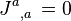$J^a{}_{,a} \, = 0$

In terms of the 4-potential (as a contravariant vector)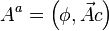$A^{a} = \left(\phi, \vec A c \right)$, where φ is the electric potential and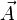$\vec A$ is the magnetic vector potential in the Lorentz gauge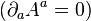$\left ( \partial_a A^a = 0 \right )$, F can be written as: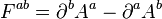$F^{ab} = \partial^b A^a - \partial^a A^b \,\!$

which leads to the 4 × 4 matrix rank-2 tensor: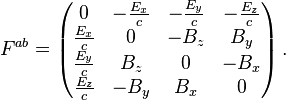$F^{ab} = \left( \begin{matrix} 0 & -\frac {E_x}{c} & -\frac {E_y}{c} & -\frac {E_z}{c} \\ \frac{E_x}{c} & 0 & -B_z & B_y \\ \frac{E_y}{c} & B_z & 0 & -B_x \\ \frac{E_z}{c} & -B_y & B_x & 0 \end{matrix} \right) .$

The fact that both electric and magnetic fields are combined into a single tensor shows the fact that, according to relativity, both of these are different parts of the same thing—by changing frames of reference, what looks like an electric field in one frame can look like a magnetic field in another frame, and the other way around.

Using the tensor form of Maxwell's equations, the first equation implies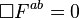$\Box F^{ab} = 0$ (See Electromagnetic four-potential for the relationship between the d'Alembertian of the four-potential and the four-current, expressed in terms of the older vector operator notation).

Different authors sometimes use different sign conventions for these tensors and 4-vectors (but this does not change what they mean).$\, F^{ab}$ and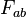$\, F_{ab}$ are not the same: they are related by the Minkowski metric tensor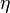$\eta$: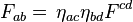$F_{ab} =\, \eta_{ac} \eta_{bd} F^{cd}$. This changes the sign of some of F's components; more complex metric dualities can be seen in general relativity.

## Images for kidsMaxwell's equations Facts for Kids. Kiddle Encyclopedia.